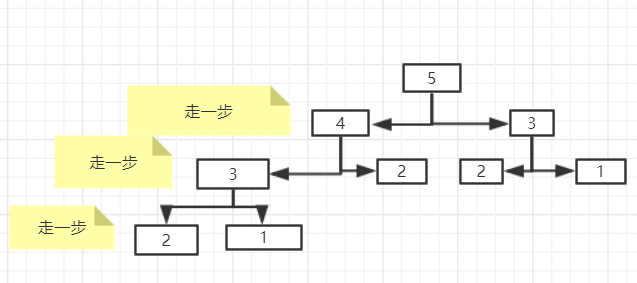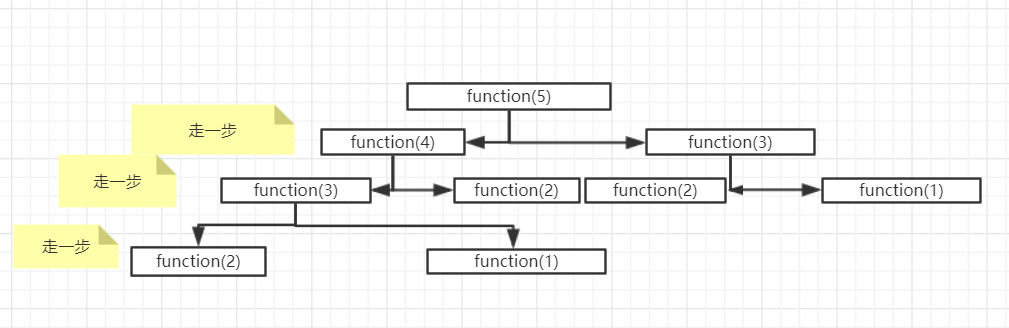• 上楼梯递归
此方法较简单但会超时。。。
题目描述 楼梯有 NN 阶，上楼可以一步上一阶，也可以一步上二阶。
编一个程序，计算共有多少种不同的走法。
输入格式 一个数字，楼梯数。
输出格式 输出走的方式总数。
样例输入
4

样例输出
5

#include <stdio.h>
#include <stdlib.h>

int step(int n)
{
int i,j;
if(n == 1)
return 1;
if(n == 2)
return 2;
return step(n-2)+step(n-1);
}

int main()
{
int n,y;
scanf("%d",&n);
y=step(n);
printf("%d",y);
return 0;
}

展开全文•  楼梯有N级台阶，上楼可以一步一级台阶，也可以一步两级台阶。编一程序，计算共有多少种不同的走法。 输入  一行，一个整数n（1 。 输出  一个整数，走法的种数。 样例输入 3 样例...
 时间限制: 1000 ms  空间限制: 262144 KB
题目描述
楼梯有N级台阶，上楼可以一步上一级台阶，也可以一步上两级台阶。编一程序，计算共有多少种不同的走法。

输入
一行，一个整数n（1 <= n <= 30），表示台阶的级数。
输出
一个整数，走法的种数。
样例输入
3 样例输出
3 方案1：走三次，每次一步 方案2：先走一级，再走两级 方案3：先走两级，再走一次

为什么是斐波拉契数列
因为你要上n阶楼梯 只有 上f(n-1) 那上一步，或者从f(n-2)那上两步
所以这样就构成了递归式。

#include <stdio.h>

typedef unsigned long long ULL;

ULL stairs(int n)
{
ULL f1=1, f2=2, temp;

if(n == 0)
return 0;
else if(n == 1)
return 1;
else if(n == 2)
return 2;

for(int i=3; i<=n; i++) {
temp = f1 + f2;
f1 = f2;
f2 = temp;
}

return f2;
}

int main(void)
{
int n;

scanf("%d", &n);

printf("%lld\n", stairs(n));

return 0;
}  


展开全文• 楼梯有n(100 > n > 0)阶台阶,上楼时可以一步1阶,也可以一步2阶,也可以一步3阶，编程计算共有多少种不同的走法。 输入 输入的每一行包括一组测试数据，即为台阶数n。最后一行为0，表示测试结束。 输出 每...
描述

楼梯有n(100 > n > 0)阶台阶,上楼时可以一步上1阶,也可以一步上2阶,也可以一步上3阶，编程计算共有多少种不同的走法。

输入

输入的每一行包括一组测试数据，即为台阶数n。最后一行为0，表示测试结束。

输出

每一行输出对应一行输入的结果，即为走法的数目。

样例输入

1

2

3

4

0

样例输出

1

2

4

7
解析：类似于斐波那契数列的递归做法。关键在于找到其递归规则。
如果是1阶楼梯，共有1种走法。
如果是两阶楼梯，共有 1 1、2两种走法。
如果是3阶楼梯，共有111、12、21、3这4种走法。
如果是4阶楼梯，可以从1阶直接上到4阶或者从2阶上到4阶或者从3阶上到4阶，共有f(1)+f(2)+f(3)种走法。
若是5阶楼梯，可分别从2阶、3阶、4阶直接上到5阶，共f(2)+f(3)+f(4)中走法。
……
所以我们得到以下递归序列：  f(n) = f(n-1) + f(n-2) + f(n-3);

//Geeksun 2018.1.13
#include <stdio.h>
#include <string.h>
int function(int step);

int main()
{
int n;
while(scanf("%d",&n)!=EOF)
{
if(n == 0)
{
break;
}
printf("%d\n",function(n));
}
return 0;
}
int function(int step)
{
if(step == 1)
{
return 1;
}
else if(step == 2)
{
return 2;
}
else if(step == 3)
{
return 4;
}
else
{
return function(step - 1) + function(step - 2) +function(step - 3);
}
}



展开全文• 从现在开始，小编正式与你一起解决爬楼梯问题 问题1 假设你现在正在爬楼梯楼梯有n级。每次你只能爬1级或者2级，那么你有多少种方法爬到楼梯的顶部？ 分析 要想解决这个问题，很明显，你有俩个解决办法，一个是模拟...
从现在开始，小编正式与你一起解决爬楼梯问题
问题1 假设你现在正在爬楼梯，楼梯有n级。每次你只能爬1级或者2级，那么你有多少种方法爬到楼梯的顶部？ 分析 要想解决这个问题，很明显，你有俩个解决办法，一个是模拟人脑处理问题的办法，动态规划法，一种是与人脑思维方式相反的方法设X法…此处烧脑中ing…(递归法） 小编重点带你研究递归的方法，动态规划法对于这道题很遗憾我想不到实现的方法。 我们以5为例这就是小编所有的走路的过程，虽然图画的有点丑，但就是那个意思，每次只能走一步或者两步。我们要想知道我们有5 个台阶，每次只能走一步或者两步的情况的总数，只需要最下面的和加起来就可以，我们现在来解决为什么下面的相加就可以。 1.一个最下面就是一个可行的方案对不对 2.只剩下一个台阶有1种可以走的办法。 ，只剩下2个台阶有两种可以走的办法。 下面附上我的程序代码
#include <iostream>
using namespace std;

int sum=0;
//n个台阶，每个台阶最多可以走4个
int function(int n){
if(n==0)

{
return 0;
}

else if(n==1)
{
sum+=1;
return 1;
}
else if(n==2)
{
sum+=2;
return 2;
}

else if(n>2)
return function(n-1)+function(n-2);
}

int main(){
int n;
cin>>n;
function(n);
cout<<sum<<endl;
return 0;
}


代码解释读懂这张图，或许你对递归有了一定的认识，这就是树。将不能解决的东西待定以下，等到树梢的时候再解决就把所有的情况都解决了。 问题2 小明参加一个大题比赛，答对了，加4分，答错或不答扣1分，有25道题，得了70分，求答对了几道。
#include <iostream>
using namespace std;

int sum=0;
int count1=0;
int count=0;-
int function(bool n)
{
if(n==true)
{
count+=1;
sum+=4;
}
else
{
sum-=1;
}
}
int main()
{

for(int a=0;a<=1;a++)
{
for(int b=0;b<=1;b++)
{
for(int c=0;c<=1;c++)
{
for(int d=0;d<=1;d++)

{
for(int e=0;e<=1;e++)
{
for(int f=0;f<=1;f++)
{
for(int g=0;g<=1;g++)
{
for(int h=0;h<=1;h++)
{
for(int i=0;i<=1;i++)
{
for(int j=0;j<=1;j++)
{
for(int k=0;k<=1;k++)
{
for(int l=0;l<=1;l++)
{
for(int m=0;m<=1;m++)
{
for(int n=0;n<=1;n++)
{
for(int o=0;o<=1;o++)
{
for(int p=0;p<=1;p++)
{
for(int q=0;q<=1;q++)
{
for(int r=0;r<=1;r++)
{
for(int s=0;s<=1;s++)
{
for(int t=0;t<=1;t++)
{
for(int u=0;u<=1;u++)
{
for(int v=0;v<=1;v++)
{
for(int w=0;w<=1;w++)
{
for(int x=0;x<=1;x++)
{    for(int y=0;y<=1;y++)
{ sum=0;
count=0;
function(a);
function(b);
function(c);
function(d);
function(e);
function(f);
function(g);
function(h);
function(i);
function(j);
function(k);
function(l);
function(m);
function(n);
function(o);
function(p);
function(q);
function(r);
function(s);
function(t);
function(u);
function(v);
function(w);
function(x);
function(y);
if(sum==70)
{
if(count!=count1)
{
cout<<count<<endl;
count1=count;
}

}

}
}
}
}
}
}
}
}
}
}
}
}
}
}
}
}
}

}
}
}
}

}
}
}
}
return 0;
}


这种算法是不是相当暴力，不过这种算法是不是你也可以有启发，比如11111100000这是11种情况，前面6种答对，后面5种答错，你就可以用这种类似的算法求值。
展开全文• 楼梯： 树老师爬楼梯，他可以每次走1级或者2级，输入楼梯的级数，求不同的走法数。 #include <stdio.h> #include <string.h> int stair(int n){ if(n==1) return 1; if(n==2) return 2; return ...c语言
• c语言函数的递归调用（汉诺塔Hanoi问题，楼梯递归问题等） 刚接触c语言的时候，感觉函数递归调用这里比较绕，难以理解，现在本着复习的目的，捋顺一下，介绍几个递归的题目。 1.什么是递归调用 函数的递归调用是指在...c语言 函数递归调用 汉诺塔问题
• * 小白上楼梯递归设计） * @author 田秀彪 * 小白正在上楼梯楼梯有n阶台阶，小白一次可以一阶，两阶或三阶， * 实现一个方法，计算小白有多少种走完楼梯的方式。 */ public class Case01_小白上楼梯 { ...算法设计
• 这题用递推最好,但是当成递归可以用来练递归 思路,第i层可以由第i-1层走一步或者i-2层走两步过来(当然,i也可以由i-2走一步再走一步过来,可是这样和前面那个i-1层走一步上来有重复) 所以f(n)=f(n-1)+f(n-2) 再设置...
• 十阶楼梯，每次可以下一阶或者两阶，有多少种方式下完十阶楼梯？ function climbStairs(n){ if(n==0){ return 1; }else if(n==2){ return 2; }else{ return climbStairs(n-1)+climbStairs(n-2); } } var a...
• * 一个楼梯有20级，每次走一级或两级，从底走到顶，一共有多少种走法 * 递归 动态规划 */ public class Floor { public static void main(String[] args) { int n=20; System.out.println(computer(n)); /...动态规划
• 楼梯：每次走1级或2级 输入：楼梯的级数 输出：不同的走法数 例 楼梯有3级，可以 每次走1级； 第一次走1级，第二次走2级； 第一次走2级，第二次走1级 共3种方法。 思路 n级台阶的走法 = 先走一级后，n-1级台阶...c++ 算法
• 小白上楼梯(递归设计) ➢小白正在上楼梯,楼梯有n阶台阶,小白一次可以1阶, 2阶或者3阶,实现一个方法,计算小白有多少种走完楼梯的方式。 import java.util.Scanner; public class Main { public static void main...
• 小白上楼梯  楼梯有n阶 一次可以1阶或者2阶或者3阶  请问小白总共有多少种上楼梯的方法？ import java.util.Scanner; public class recursion1 { public static void main(String[] args) { // TODO Auto-...
• 小白上楼梯递归实现）题目描述输入输出实现思路代码实现 题目描述 小白正在上楼梯楼梯有n阶台阶，小白一次可以1阶，2阶，3阶。 实现一个方法，计算小白有多少种上楼梯的方式。 输入输出 输出：台阶数量。 输出...算法 c++ c语言
• 楼梯的算法总结 (1).一次可以走一阶或两阶 (2).一次可以走一阶或两阶或三阶 (3)....两种实现方式 (1).递归 (2)....（1）递归的思想：就是一...一层递归调用结束后会返回给一层， 依次类推，等到压栈的函数全部出栈递...
• 超级楼梯 Time Limit: 2000/1000 MS (Java/Others) Memory Limit: 65536/32768 K (Java/Others) Total Submission(s): 37808 Accepted Submission(s): 19442 Problem Description 有一楼梯共M级，刚开始...
• 小白正在上楼梯楼梯有n阶台阶，小白一次可以1阶，2阶或者3阶，实现一个方法,计算小白有多少种走完楼梯的方式。 提示： 设n阶台阶的走法数为f(n)。如果只有1个台阶，走法有1种（一步1个台阶），即f(1)=1；如果...
• 今天分享一道字节跳动的面试题：爬楼梯Ⅱ 。 题目描述： 一个台阶总共有 n 级，如果一次可以跳 1 级，也可以跳 2 级，但不能连续两次跳 2 级。求总共有多少总跳法。 分析： 如果没有“不能连续两次跳 2 级”的约束，...
• #include <iostream> using namespace std; int N; int stairs(int n){ //递归出口1 if( n < 0) return 0; //递归出口2 if( n == 1) return 1; return stairs(n-1) + stairs(n...
•java 优化
• 有一楼梯共M级，刚开始时你在第一级，若每次只能跨一级或二级，要走第M级，共有多少种走法？ Input 输入数据首先包含一个整数N，表示测试实例的个数，然后是N行数据，每行包含一个整数M（1<=M<=40）,...
• 假设楼梯有N阶，一次只能爬一阶或两阶，问有几种爬楼梯的方法？ N=1， 1种 N=2， 2种 N=3， 3种 N=4， 5种 N=5， 8种 发现这好像斐波那契数列，后一个数等于前两个数之和。 F(N+2)=F(N+1)+F(N) 下面是c++代码： #......